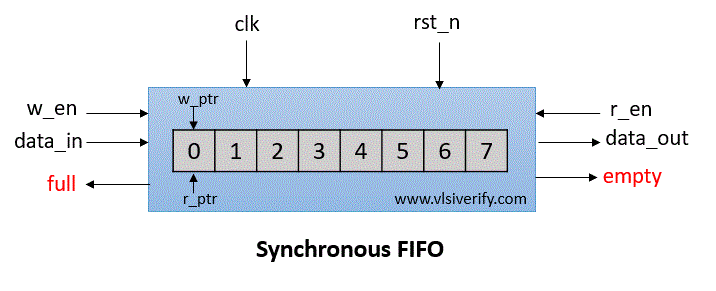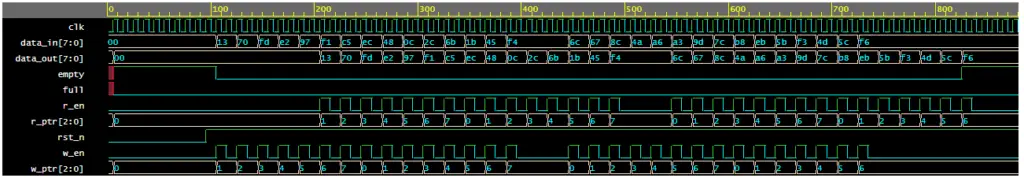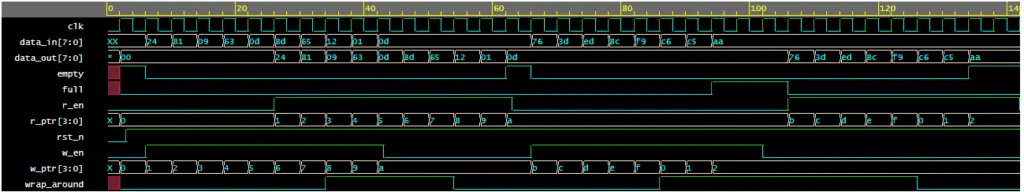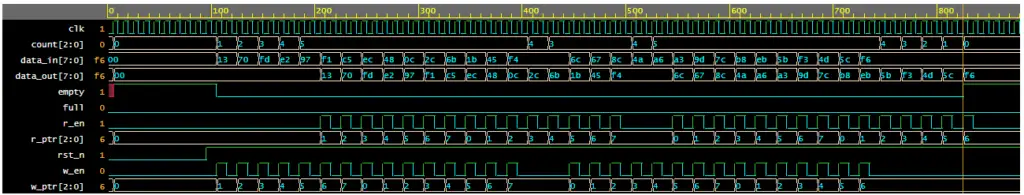Tutorials

First In First Out (FIFO) is a very popular and useful design block for purpose of synchronization and a handshaking mechanism between the modules.

Depth of FIFO: The number of slots or rows in FIFO is called the depth of the FIFO.

Width of FIFO: The number of bits that can be stored in each slot or row is called the width of the FIFO.

There are two types of FIFOs

1. Synchronous FIFO
2. Asynchronous FIFO

Synchronous FIFO

In Synchronous FIFO, data read and write operations use the same clock frequency. Usually, they are used with high clock frequency to support high-speed systems.## Synchronous FIFO Operation

Signals:

wr_en: write enable

wr_data: write data

full: FIFO is full

empty: FIFO is empty

w_ptr: write pointer

### FIFO write operation

FIFO can store/write the wr_data at every posedge of the clock based on wr_en signal till it is full. The write pointer gets incremented on every data write in FIFO memory.

The data can be taken out or read from FIFO at every posedge of the clock based on the rd_en signal till it is empty. The read pointer gets incremented on every data read from FIFO memory.

## Synchronous FIFO Verilog Code

A synchronous FIFO can be implemented in various ways. Full and empty conditions differ based on implementation.

### Method 1

In this method, the width of the write and read pointer = log2(depth of FIFO). The FIFO full and empty conditions can be determined as

#### Empty condition

w_ptr == r_ptr i.e. write and read pointers has the same value.

#### Full condition

The full condition means every slot in the FIFO is occupied, but then w_ptr and r_ptr will again have the same value. Thus, it is not possible to determine whether it is a full or empty condition. Thus, the last slot of FIFO is intentionally kept empty, and the full condition can be written as (w_ptr+1’b1) == r_ptr)

#### Verilog Code

``````module synchronous_fifo #(parameter DEPTH=8, DATA_WIDTH=8) (
input clk, rst_n,
input w_en, r_en,
input [DATA_WIDTH-1:0] data_in,
output reg [DATA_WIDTH-1:0] data_out,
output full, empty
);

reg [\$clog2(DEPTH)-1:0] w_ptr, r_ptr;
reg [DATA_WIDTH-1:0] fifo[DEPTH];

// Set Default values on reset.
always@(posedge clk) begin
if(!rst_n) begin
w_ptr <= 0; r_ptr <= 0;
data_out <= 0;
end
end

// To write data to FIFO
always@(posedge clk) begin
if(w_en & !full)begin
fifo[w_ptr] <= data_in;
w_ptr <= w_ptr + 1;
end
end

// To read data from FIFO
always@(posedge clk) begin
if(r_en & !empty) begin
data_out <= fifo[r_ptr];
r_ptr <= r_ptr + 1;
end
end

assign full = ((w_ptr+1'b1) == r_ptr);
assign empty = (w_ptr == r_ptr);
endmodule``````

#### Testbench Code - 1

``````module sync_fifo_TB;
parameter DATA_WIDTH = 8;

reg clk, rst_n;
reg w_en, r_en;
reg [DATA_WIDTH-1:0] data_in;
wire [DATA_WIDTH-1:0] data_out;
wire full, empty;

// Queue to push data_in
reg [DATA_WIDTH-1:0] wdata_q[\$], wdata;

synchronous_fifo s_fifo(clk, rst_n, w_en, r_en, data_in, data_out, full, empty);

always #5ns clk = ~clk;

initial begin
clk = 1'b0; rst_n = 1'b0;
w_en = 1'b0;
data_in = 0;

repeat(10) @(posedge clk);
rst_n = 1'b1;

repeat(2) begin
for (int i=0; i<30; i++) begin
@(posedge clk);
w_en = (i%2 == 0)? 1'b1 : 1'b0;
if (w_en & !full) begin
data_in = \$urandom;
wdata_q.push_back(data_in);
end
end
#50;
end
end

initial begin
clk = 1'b0; rst_n = 1'b0;
r_en = 1'b0;

repeat(20) @(posedge clk);
rst_n = 1'b1;

repeat(2) begin
for (int i=0; i<30; i++) begin
@(posedge clk);
r_en = (i%2 == 0)? 1'b1 : 1'b0;
if (r_en & !empty) begin
#1;
wdata = wdata_q.pop_front();
if(data_out !== wdata) \$error("Time = %0t: Comparison Failed: expected wr_data = %h, rd_data = %h", \$time, wdata, data_out);
else \$display("Time = %0t: Comparison Passed: wr_data = %h and rd_data = %h",\$time, wdata, data_out);
end
end
#50;
end

\$finish;
end

initial begin
\$dumpfile("dump.vcd"); \$dumpvars;
end
endmodule
``````

Output:

``````Time = 206: Comparison Passed: wr_data = 13 and rd_data = 13
Time = 226: Comparison Passed: wr_data = 70 and rd_data = 70
Time = 246: Comparison Passed: wr_data = fd and rd_data = fd
Time = 266: Comparison Passed: wr_data = e2 and rd_data = e2
Time = 286: Comparison Passed: wr_data = 97 and rd_data = 97
Time = 306: Comparison Passed: wr_data = f1 and rd_data = f1
Time = 326: Comparison Passed: wr_data = c5 and rd_data = c5
Time = 346: Comparison Passed: wr_data = ec and rd_data = ec
Time = 366: Comparison Passed: wr_data = 48 and rd_data = 48
Time = 386: Comparison Passed: wr_data = 0c and rd_data = 0c
Time = 406: Comparison Passed: wr_data = 2c and rd_data = 2c
Time = 426: Comparison Passed: wr_data = 6b and rd_data = 6b
Time = 446: Comparison Passed: wr_data = 1b and rd_data = 1b
Time = 466: Comparison Passed: wr_data = 45 and rd_data = 45
Time = 486: Comparison Passed: wr_data = f4 and rd_data = f4
Time = 546: Comparison Passed: wr_data = 6c and rd_data = 6c
Time = 566: Comparison Passed: wr_data = 67 and rd_data = 67
Time = 586: Comparison Passed: wr_data = 8c and rd_data = 8c
Time = 606: Comparison Passed: wr_data = 4a and rd_data = 4a
Time = 626: Comparison Passed: wr_data = a6 and rd_data = a6
Time = 646: Comparison Passed: wr_data = a3 and rd_data = a3
Time = 666: Comparison Passed: wr_data = 9d and rd_data = 9d
Time = 686: Comparison Passed: wr_data = 7c and rd_data = 7c
Time = 706: Comparison Passed: wr_data = b8 and rd_data = b8
Time = 726: Comparison Passed: wr_data = eb and rd_data = eb
Time = 746: Comparison Passed: wr_data = 5b and rd_data = 5b
Time = 766: Comparison Passed: wr_data = f3 and rd_data = f3
Time = 786: Comparison Passed: wr_data = 4d and rd_data = 4d
Time = 806: Comparison Passed: wr_data = 5c and rd_data = 5c
Time = 826: Comparison Passed: wr_data = f6 and rd_data = f6``````

#### Waveform#### Testbench Code - 2

``````module sync_fifo_TB;
reg clk, rst_n;
reg w_en, r_en;
reg [7:0] data_in;
wire [7:0] data_out;
wire full, empty;

synchronous_fifo s_fifo(clk, rst_n, w_en, r_en, data_in, data_out, full, empty);

always #2 clk = ~clk;
initial begin
clk = 0; rst_n = 0;
w_en = 0; r_en = 0;
#3 rst_n = 1;
drive(20);
drive(40);
\$finish;
end

if(!full) begin
w_en = 1;
data_in = \$random;
#1 \$display("Push In: w_en=%b, r_en=%b, data_in=%h",w_en, r_en,data_in);
end
else \$display("FIFO Full!! Can not push data_in=%d", data_in);

if(!empty) begin
r_en = 1;
#1 \$display("Pop Out: w_en=%b, r_en=%b, data_out=%h",w_en, r_en,data_out);
end
else \$display("FIFO Empty!! Can not pop data_out");

w_en = 0; r_en = 0;
fork
begin
repeat(10) begin @(posedge clk) push(); end
w_en = 0;
end
begin
#delay;
repeat(10) begin @(posedge clk) pop(); end
r_en = 0;
end
join

initial begin
\$dumpfile("dump.vcd"); \$dumpvars;
end
endmodule``````

### Method 2

In order to avoid an empty slot as mentioned in method 1, the width of write and read pointers is increased by 1 bit. This extra bit helps determine empty and full conditions when FIFO is empty (w_ptr == r_ptr when all slots are empty) and FIFO is full (w_ptr == r_ptr when all slots are full).

#### Empty condition

w_ptr == r_ptr i.e. write and read pointers has the same value. MSB of w_ptr and r_ptr also has the same value.

#### Full condition

w_ptr == r_ptr i.e. write and read pointers has the same value, but the MSB of w_ptr and r_ptr differs.

#### Verilog Code with an extra bit in write/read pointers

``````module synchronous_fifo #(parameter DEPTH=8, DATA_WIDTH=8) (
input clk, rst_n,
input w_en, r_en,
input [DATA_WIDTH-1:0] data_in,
output reg [DATA_WIDTH-1:0] data_out,
output full, empty
);

parameter PTR_WIDTH = \$clog2(DEPTH);
reg [PTR_WIDTH:0] w_ptr, r_ptr; // addition bit to detect full/empty condition
reg [DATA_WIDTH-1:0] fifo[DEPTH];
reg wrap_around;

// Set Default values on reset.
always@(posedge clk) begin
if(!rst_n) begin
w_ptr <= 0; r_ptr <= 0;
data_out <= 0;
end
end

// To write data to FIFO
always@(posedge clk) begin
if(w_en & !full)begin
fifo[w_ptr[PTR_WIDTH-1:0]] <= data_in;
w_ptr <= w_ptr + 1;
end
end

// To read data from FIFO
always@(posedge clk) begin
if(r_en & !empty) begin
data_out <= fifo[r_ptr[PTR_WIDTH-1:0]];
r_ptr <= r_ptr + 1;
end
end

assign wrap_around = w_ptr[PTR_WIDTH] ^ r_ptr[PTR_WIDTH]; // To check MSB of write and read pointers are different

//Full condition: MSB of write and read pointers are different and remainimg bits are same.
assign full = wrap_around & (w_ptr[PTR_WIDTH-1:0] == r_ptr[PTR_WIDTH-1:0]);

//Empty condition: All bits of write and read pointers are same.
//assign empty = !wrap_around & (w_ptr[PTR_WIDTH-1:0] == r_ptr[PTR_WIDTH-1:0]);
//or
assign empty = (w_ptr == r_ptr);
endmodule``````

Output:

``````Push In: w_en=1, r_en=0, data_in=24
Push In: w_en=1, r_en=0, data_in=81
Push In: w_en=1, r_en=0, data_in=09
Push In: w_en=1, r_en=0, data_in=63
Push In: w_en=1, r_en=0, data_in=0d
Push In: w_en=1, r_en=1, data_in=8d
Pop Out: w_en=1, r_en=1, data_out=24
Push In: w_en=1, r_en=1, data_in=65
Pop Out: w_en=1, r_en=1, data_out=81
Push In: w_en=1, r_en=1, data_in=12
Pop Out: w_en=1, r_en=1, data_out=09
Push In: w_en=1, r_en=1, data_in=01
Pop Out: w_en=1, r_en=1, data_out=63
Push In: w_en=1, r_en=1, data_in=0d
Pop Out: w_en=0, r_en=1, data_out=0d
Pop Out: w_en=0, r_en=1, data_out=8d
Pop Out: w_en=0, r_en=1, data_out=65
Pop Out: w_en=0, r_en=1, data_out=12
Pop Out: w_en=0, r_en=1, data_out=01
Pop Out: w_en=0, r_en=1, data_out=0d
Push In: w_en=1, r_en=0, data_in=76
Push In: w_en=1, r_en=0, data_in=3d
Push In: w_en=1, r_en=0, data_in=ed
Push In: w_en=1, r_en=0, data_in=8c
Push In: w_en=1, r_en=0, data_in=f9
Push In: w_en=1, r_en=0, data_in=c6
Push In: w_en=1, r_en=0, data_in=c5
Push In: w_en=1, r_en=0, data_in=aa
FIFO Full!! Can not push data_in=170
FIFO Full!! Can not push data_in=170
Pop Out: w_en=0, r_en=1, data_out=76
Pop Out: w_en=0, r_en=1, data_out=3d
Pop Out: w_en=0, r_en=1, data_out=ed
Pop Out: w_en=0, r_en=1, data_out=8c
Pop Out: w_en=0, r_en=1, data_out=f9
Pop Out: w_en=0, r_en=1, data_out=c6
Pop Out: w_en=0, r_en=1, data_out=c5
Pop Out: w_en=0, r_en=1, data_out=aa
FIFO Empty!! Can not pop data_out
FIFO Empty!! Can not pop data_out``````

#### Waveform### Method 3

The synchronous FIFO can also be implemented using a common counter that can be incremented or decremented based on write to the FIFO or read from the FIFO respectively.

#### Empty condition

count == 0 i.e. FIFO contains nothing.

#### Full condition

count == FIFO_DEPTH i.e. counter value has reached till the depth of FIFO

#### Verilog Code using counter

``````module synchronous_fifo #(parameter DEPTH=8, DATA_WIDTH=8) (
input clk, rst_n,
input w_en, r_en,
input [DATA_WIDTH-1:0] data_in,
output reg [DATA_WIDTH-1:0] data_out,
output full, empty
);

reg [\$clog2(DEPTH)-1:0] w_ptr, r_ptr;
reg [DATA_WIDTH-1:0] fifo[DEPTH];
reg [\$clog2(DEPTH)-1:0] count;

// Set Default values on reset.
always@(posedge clk) begin
if(!rst_n) begin
w_ptr <= 0; r_ptr <= 0;
data_out <= 0;
count <= 0;
end
else begin
case({w_en,r_en})
2'b00, 2'b11: count <= count;
2'b01: count <= count - 1'b1;
2'b10: count <= count + 1'b1;
endcase
end
end

// To write data to FIFO
always@(posedge clk) begin
if(w_en & !full)begin
fifo[w_ptr] <= data_in;
w_ptr <= w_ptr + 1;
end
end

// To read data from FIFO
always@(posedge clk) begin
if(r_en & !empty) begin
data_out <= fifo[r_ptr];
r_ptr <= r_ptr + 1;
end
end

assign full = (count == DEPTH);
assign empty = (count == 0);
endmodule``````

Output:

``````Time = 206: Comparison Passed: wr_data = 13 and rd_data = 13
Time = 226: Comparison Passed: wr_data = 70 and rd_data = 70
Time = 246: Comparison Passed: wr_data = fd and rd_data = fd
Time = 266: Comparison Passed: wr_data = e2 and rd_data = e2
Time = 286: Comparison Passed: wr_data = 97 and rd_data = 97
Time = 306: Comparison Passed: wr_data = f1 and rd_data = f1
Time = 326: Comparison Passed: wr_data = c5 and rd_data = c5
Time = 346: Comparison Passed: wr_data = ec and rd_data = ec
Time = 366: Comparison Passed: wr_data = 48 and rd_data = 48
Time = 386: Comparison Passed: wr_data = 0c and rd_data = 0c
Time = 406: Comparison Passed: wr_data = 2c and rd_data = 2c
Time = 426: Comparison Passed: wr_data = 6b and rd_data = 6b
Time = 446: Comparison Passed: wr_data = 1b and rd_data = 1b
Time = 466: Comparison Passed: wr_data = 45 and rd_data = 45
Time = 486: Comparison Passed: wr_data = f4 and rd_data = f4
Time = 546: Comparison Passed: wr_data = 6c and rd_data = 6c
Time = 566: Comparison Passed: wr_data = 67 and rd_data = 67
Time = 586: Comparison Passed: wr_data = 8c and rd_data = 8c
Time = 606: Comparison Passed: wr_data = 4a and rd_data = 4a
Time = 626: Comparison Passed: wr_data = a6 and rd_data = a6
Time = 646: Comparison Passed: wr_data = a3 and rd_data = a3
Time = 666: Comparison Passed: wr_data = 9d and rd_data = 9d
Time = 686: Comparison Passed: wr_data = 7c and rd_data = 7c
Time = 706: Comparison Passed: wr_data = b8 and rd_data = b8
Time = 726: Comparison Passed: wr_data = eb and rd_data = eb
Time = 746: Comparison Passed: wr_data = 5b and rd_data = 5b
Time = 766: Comparison Passed: wr_data = f3 and rd_data = f3
Time = 786: Comparison Passed: wr_data = 4d and rd_data = 4d
Time = 806: Comparison Passed: wr_data = 5c and rd_data = 5c
Time = 826: Comparison Passed: wr_data = f6 and rd_data = f6``````

#### WaveformVerilog Codes